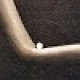You are currently browsing the tag archive for the ‘trace formula’ tag.

Atle Selberg, who made immense and fundamental contributions to analytic number theory and related areas of mathematics, died last Monday, aged 90.

Selberg’s early work was focused on the study of the Riemann zeta function$\zeta(s)$. In 1942, Selberg showed that a positive fraction of the zeroes of this function lie on the critical line$\hbox{Re}(s)=1/2$. Apart from improvements in the fraction (the best value currently being a little over 40%, a result of Conrey), this is still one of the strongest partial results we have towards the Riemann hypothesis. (I discuss Selberg’s result, and the method of mollifiers he introduced there, in a little more detail after the jump.)

In working on the zeta function, Selberg developed two powerful tools which are still used routinely in analytic number theory today. The first is the method of mollifiers to smooth out the magnitude oscillations of the zeta function, making the (more interesting) phase oscillation more visible. The second was the method of the Selberg$\Lambda^2$ sieve, which is a particularly elegant choice of sieve which allows one to count patterns in almost primes (and hence to upper bound patterns in primes) quite accurately. Variants of the Selberg sieve were a crucial ingredient in, for instance, the recent work of Goldston-Yıldırım-Pintz on prime gaps, as well as the work of Ben Green and myself on arithmetic progressions in primes. (I discuss the Selberg sieve, as well as the Selberg symmetry formula below, in my post on the parity problem. Incidentally, Selberg was the first to formalise this problem as a significant obstruction in sieve theory.)

For all of these achievements, Selberg was awarded the Fields Medal in 1950. Around that time, Selberg and Erdős also produced the first elementary proof of the prime number theorem. A key ingredient here was the Selberg symmetry formula, which is an elementary analogue of the prime number theorem for almost primes.

But perhaps Selberg’s greatest contribution to mathematics was his discovery of the Selberg trace formula, which is a non-abelian generalisation of the Poisson summation formula, and which led to many further deep connections between representation theory and number theory, and in particular being one of the main inspirations for the Langlands program, which in turn has had an impact on many different parts of mathematics (for instance, it plays a role in Wiles’ proof of Fermat’s last theorem). For an introduction to the trace formula, its history, and its impact, I recommend the survey article of Arthur.

Other major contributions of Selberg include the Rankin-Selberg theory connecting Artin L-functions from representation theory to the integrals of automorphic forms (very much in the spirit of the Langlands program), and the Chowla-Selberg formula relating the Gamma function at rational values to the periods of elliptic curves with complex multiplication. He also made an influential conjecture on the spectral gap of the Laplacian on quotients of$SL(2,{\Bbb R})$ by congruence groups, which is still open today (Selberg had the first non-trivial partial result). As an example of this conjecture’s impact, Selberg’s eigenvalue conjecture has inspired some recent work of Sarnak-Xue, Gamburd, and Bourgain-Gamburd on new constructions of expander graphs, and has revealed some further connections between number theory and arithmetic combinatorics (such as sum-product theorems); see this announcement of Bourgain-Gamburd-Sarnak for the most recent developments (this work, incidentally, also employs the Selberg sieve). As observed by Satake, Selberg’s eigenvalue conjecture and the more classical Ramanujan-Petersson conjecture can be unified into a single conjecture, now known as the Ramanujan-Selberg conjecture; the eigenvalue conjecture is then essentially an archimedean (or “non-dyadic“) special case of the general Ramanujan-Selberg conjecture. (The original (dyadic) Ramanujan-Petersson conjecture was finally proved by Deligne-Serre, after many important contributions by other authors, but the non-dyadic version remains open.)

 Dan Romik on the Rie… on Polymath15, eleventh thread: W…Andrew Verras on Analysis IIYaver Gulusoy (cebis… on Analysis IIAnonymous on Don’t prematurely obsess… What do mathematicia… on The Collatz conjecture, Little…L on Don’t prematurely obsess…L on Don’t prematurely obsess…Andrew Krause on Don’t prematurely obsess…Arsene1412 on Living Proof: Stories of Resil…Anonymous on Don’t prematurely obsess…Yaver Gulusoy (cebis… on Analysis IIAnonymous on Analysis IMath grad student on Work hardNic Dobson on How to assign partial credit o…Nic Dobson on How to assign partial credit o…